# Simple interest And Compound interest for RRB PO & RRB Clerk – Quiz 7

## Simple interest And Compound interest for RRB PO & RRB Clerk – Quiz 7

Simple interest And Compound interest plays a significant role in Quantitative Aptitude Section of banking exams such as IBPS, SBI and RBI PO and Clerk. You will get at least 1-2 questions from Simple interest And Compound interest in one of IBPS, SBI and RBI PO & clerk exam. So, aspirants should focus on Simple interest And Compound interest questions in detail. Here, we are providing you with the Simple interest And Compound interest questions quiz with the detailed solution so that you can easily prepare for Simple interest And Compound interest questions. We are providing here all-important latest pattern-based questions and Previous Year Questions of Simple interest And Compound interest of various Government Exam like IBPS, SBI, and RBI PO and Clerk exam. This Simple interest And Compound interest quiz we are providing is free. Attempt this Simple interest And Compound interest quiz to practice important questions with answers and solutions. And score better in IBPS, SBI and RBI PO and Clerk exam.

1. A man gets a simple interest of Rs. 1000 on a certain principal at the rate of 5 p.c.p.a. in 4 years. What compound interest will the man get on twice the principal in two years at the same rate?
(a) Rs. 1050
(b) Rs. 1005
(c) Rs. 11025
(d) Rs. 10125
(e) Rs. 102502. Mayank borrowed Rs. 48000 from a bank at 25/2 % per annum compound interest at the end of 1st, 2nd and 3rd year, he paid 14000, 13000 and 16000 respectively. If he wanted to clear his loan at the end of 4th year, what would he pay at the end of the fourth year to clear his loan ?
(a) 21000
(b) 22500
(c) 16800
(d) 26000
(e) None of these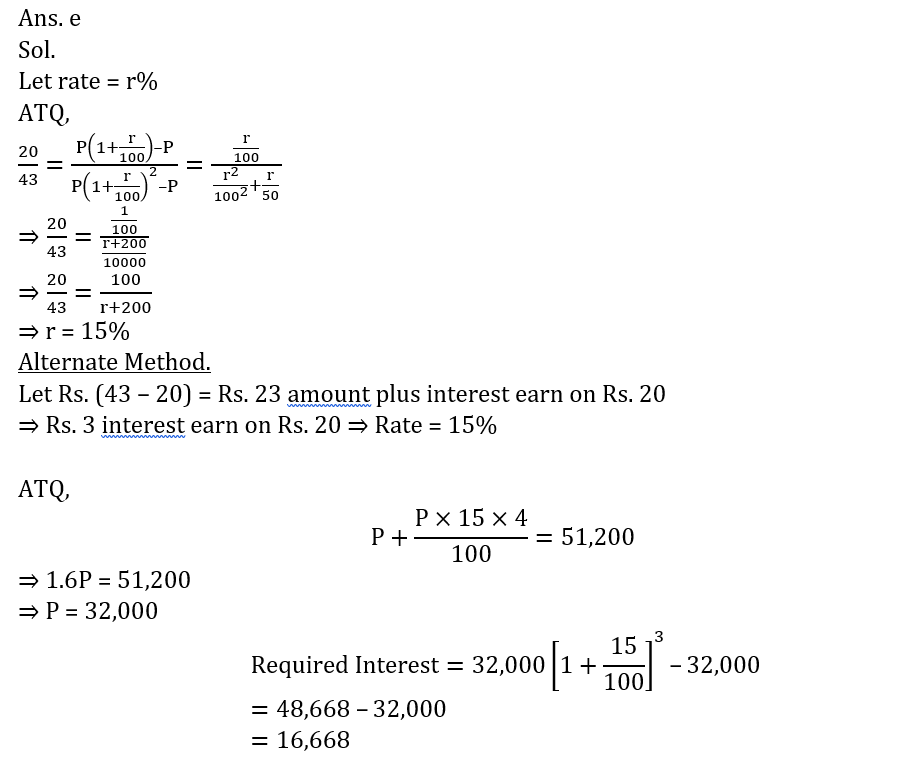3. A certain sum of money becomes thrice of itself in 4 years on SI. Find the time period in which it becomes 8 times of itself?
(a) 7 yrs
(b) 14 yrs
(c) 6 yrs
(d) 12 yrs
(e) 28 yrs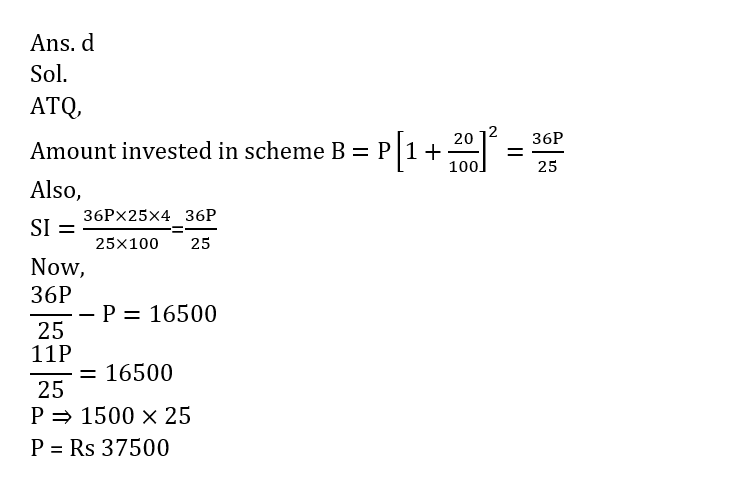4. Karan invested a sum of money ‘P’ for two years in scheme A at 20% p.a. CI. The amount received at end of two years from scheme A, reinvested in scheme B for 4 years that offers 25% p.a. S.I. If total interest received from scheme B is Rs 16500 more than P, then find ‘P’?
(a) Rs 35500
(b) Rs 27500
(c) Rs 34500
(d) Rs 37500
(e) Rs. 32500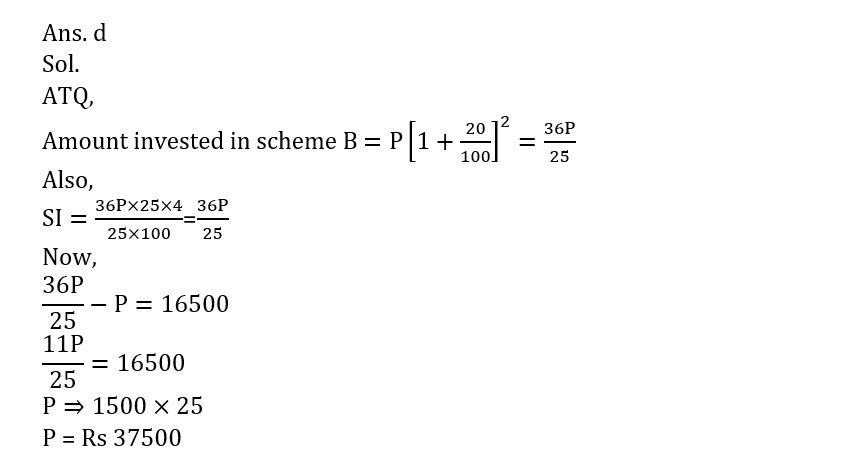5. Find ratio between S.I. and C.I. on a sum of money invested for 3 years at 5% rate of interest per annum?
(a) 15 : 1261
(b) 151 : 156
(c) 121 : 441
(d) 1200 : 1261
(e) 121 : 4846. Find time in which a sum of money becomes 8 times of itself if it becomes triple in 2 years p.a S.I ?
(a) 8 yrs
(b) 7 yrs
(c) 5 yrs
(d) 4 yrs
(e) 6 yrs7. A man invested a sum in a scheme ‘P’ which offer 10% C.I.P.A. for 3 years and earn Rs. 1655. Find the interest earn by man, if he invested total amount he gets from scheme ‘P’, in scheme Q which offer 8% S.I.P.A for 5 years.
(a) Rs.4317
(b) Rs.1655
(c) Rs.1331
(d) Rs.2662
(e) Rs.2000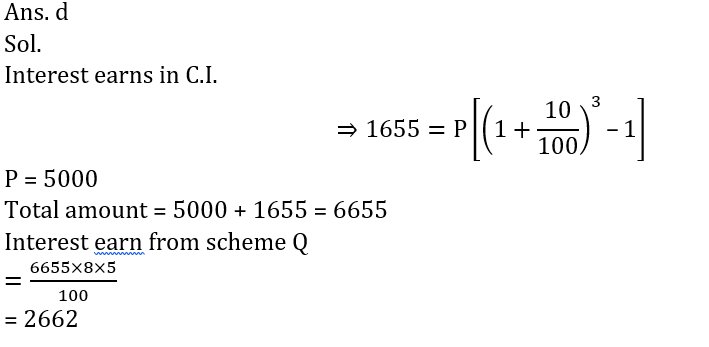8. The interest earned when Rs P is invested for four years in a scheme offering 9% p.a. simple interest is more than the interest earned when the same sum (Rs P) is invested for two years in another scheme offering 12% p.a. simple interest, by Rs 360. What is the value of P?
(a) 2000
(b) 3500
(c) 2500
(d) 4000
(e) 3000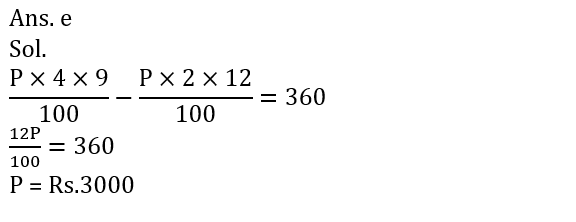9. Raman invested Rs. P in scheme ‘X’ which offers some rate at compound interest p.a. If ratio of interest earned by him after first year to second year from scheme ‘X’ is 20 : 43 while if Raman invested Rs. P in scheme ‘Q’ which offers same rate but at simple interest then after 4 years sum amounts to Rs. 51,200, then find the interest he can earn if he invested Rs. P for 3 years in scheme ‘X’.
(a) 16,248
(b) 16,868
(c) 15,548
(d) 14,656
(e) 16,66810. P invested Rs. X in a scheme for 2 year which offered simple at the rate of 15% per annum and Q invested Rs. (X + 2500) in another scheme for same period of time, which offered compound interest at the rate of 20% per annum. If from both scheme P and Q got total interest of Rs. 32550, then find the value of X ?
(a) 41500
(b) 42500
(c) 40500
(d) 40000
(e) 38250#### Attempt Quantitative Aptitude Topic Wise Online Test Series

Recommended PDF’s for 2021:

### 2021 Preparation Kit PDF

#### Most important PDF’s for Bank, SSC, Railway and Other Government Exam : Download PDF Now

AATMA-NIRBHAR Series- Static GK/Awareness Practice Ebook PDF Get PDF here
The Banking Awareness 500 MCQs E-book| Bilingual (Hindi + English) Get PDF here
AATMA-NIRBHAR Series- Banking Awareness Practice Ebook PDF Get PDF here
Computer Awareness Capsule 2.O Get PDF here
AATMA-NIRBHAR Series Quantitative Aptitude Topic-Wise PDF 2020 Get PDF here
Memory Based Puzzle E-book | 2016-19 Exams Covered Get PDF here
Caselet Data Interpretation 200 Questions Get PDF here
Puzzle & Seating Arrangement E-Book for BANK PO MAINS (Vol-1) Get PDF here
ARITHMETIC DATA INTERPRETATION 2.O E-book Get PDF here
3